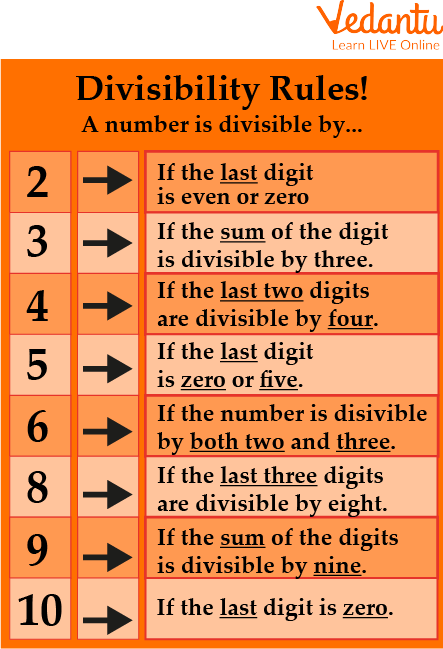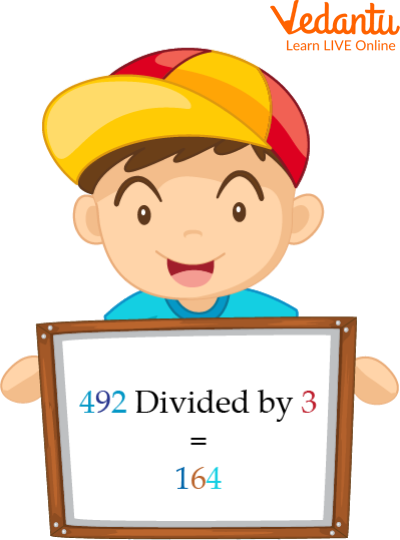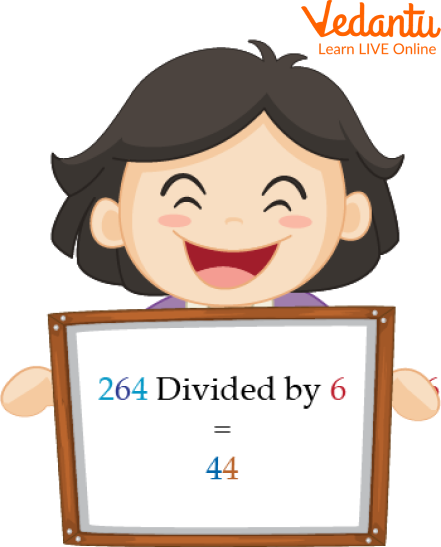Courses
Courses for Kids
Free study material
Free LIVE classes
More

# Divisibility RulesLIVE
Join Vedantu’s FREE Mastercalss

## An Overview of the Divisibility Rule

Do you know how to quickly answer whether a number is divisible by another number, just by looking at it? Yes, using divisibility rules, one can quickly answer whether a number is divisible by another number or not. The below article covers the topic of divisibility rules, deeply by discussing some divisibility rules practice problems. Reading this article, students will be able to easily find the divisors of a given number. Let us now start learning about this topic in detail in this article.

## What do You Mean by Divisibility Rules?

The divisibility rules were outlined by the famous mathematician Martin Gardner in the year 1962. These serve as a convenient shorthand for checking if an integer is divisible by a given set of divisors without actually dividing, typically by looking at the digits of the number.

## Divisibility Rules for the Numbers 2 to 10

1. The Divisibility Rule for 2: Check whether the last digit of a given number is zero or even number. If yes, then the given number is divisible by 2.

2. The Divisibility Rule for 3: Check whether the sum of the digits of a given number is divisible by 3 or not. If so, then the given number is divisible by 3.

3. The Divisibility Rule for 4: Check whether the last two digits of a given number are divisible by 4 or not. If yes, then the given number is divisible by 4.

4. The Divisibility Rule for 5: If the last digits of a number are 0 or 5, then the number is divisible by 5, otherwise not.

5. The Divisibility Rule for 6: Check whether the given number is divisible by 2 and 3 both or not. If yes, then the given number is divisible by 6.

6. The Divisibility Rule for 7: To check whether the given number is divisible by 7, one must multiply the last digit by 2, and then subtract the product from the number being left. Finally, if the difference obtained is 0 or a multiple of 7, then the given number is divisible by 7.

7. The Divisibility Rule for 8: Check whether the last three digits of the given number are divisible by 8 or not. If yes, then the given number is divisible by 8.

8. The Divisibility Rule for 9: Check whether the sum of the digits of a given number is divisible by 9 or not. If yes, then the given number is divisible by 9.

9. The Divisibility Rule for 10: If the last digit of a number is zero, then the given number is divisible by 10, otherwise not.Divisibility Rules

## Solved Problems on Divisibility Rules

1. Find whether 492 is divisible by 3 or not.

Ans: Steps to be followed to solve the divisibility problem are given:

• Write down the original number, 492.

• Add the individual digit of the number, 492, i.e., 4 + 9 + 2 = 15.

• Check whether 15 is divisible by 3 or not. If yes, then the given number is divisible by 3 otherwise not.

• As 15 is divisible by 3, we can conclude that the number is divisible by 3.

Hence, the given number 492 is divisible by 3.Problems on Divisibility Rules

2. Check whether 6 divides 264 or not.

Ans: Steps to be followed to solve the divisibility problem are given:

• Write down the original number 264.

• Now, find the prime factors of 6, i.e., 2 and 3.

• To check whether the given number is divisible by 6 or not, one must check its divisibility with both 2 and 3.

• As the last digit of the number 264 is even, so it is divisible by 2.

• Now, add the individual digit of the number, 264, i.e., 2 + 6 + 4 = 12. As 12 is divisible by 3, so 264 is divisible by 3.

• The provided number is divisible by both 2 and 3, so it is divisible by 6.

Hence, the given number, 264, is divisible by 6.Problems on Divisibility Rules

3. Check whether 7 divides 231 or not.

Ans: Steps to be followed to solve the divisibility problem are given below:

• Multiply the last digit of the number 231 by 2, i.e., 1×2.

• Now subtract the product, 2 from the number being left, i.e., 23.

• The difference obtained is 21 which is a multiple of 7.

Thus, the given number, 231, is divisible by 7.

## Divisibility Rule Practice Problems

Some of the divisibility rule practice problems are given below, which need to be solved by the students themselves.

1. Check the divisibility of 4 for the number 864.

Ans: The given number is divisible by 4.

2. Does 5 divide the number 46825 leaving no remainder?

Ans. Yes.

3. Without actual division, find whether 35278 is divisible by 6 or not.

Ans: No.

4. Explain the divisibility rule of 7.

## Aptitude Questions on Divisibility

1. Is 414 divisible by 6? Check using the divisibility rule of 6.

Ans: Yes.

2. How many of the following numbers are divisible by 132?

264, 396, 462, 792, 968, 2178, 5184, 6336

Ans: Only four numbers are divisible by 132, i.e., 264, 396, 792, and 6336.

3. Is 399 divisible by 7?

Ans: Yes.

## Summary

These rules serve as the most important tool in Mathematics to solve any problem involving division. Every topic in this article has been discussed to the point in a simple language and attractive style for better clarity of the concepts. Some Aptitude Questions on Divisibility are also given along with their answers to make students aware of the pattern.

Last updated date: 27th Sep 2023
Total views: 96.3k
Views today: 2.96k

## FAQs on Divisibility Rules

1. What is division?

Division is an arithmetic operation that is used to divide two numbers. Generally, the symbol that depicts the division is '÷' or '/'. The number being divided is called the dividend, and the number which is used to divide the dividend is called the divisor.

2. What is the value of x when 42x is completely divisible by 5?

Using the divisibility rule of 5, we can conclude that a number is divisible by 5 only if its last digit is 0 or 5, i.e., x must be 0 or 5 for this number to be divisible by 5.

3. Explain the divisibility rule of 3.

The Divisibility Rule for 3: Check whether the sum of the digits of a given number is divisible by 3 or not. If so, then the given number is divisible by 3.